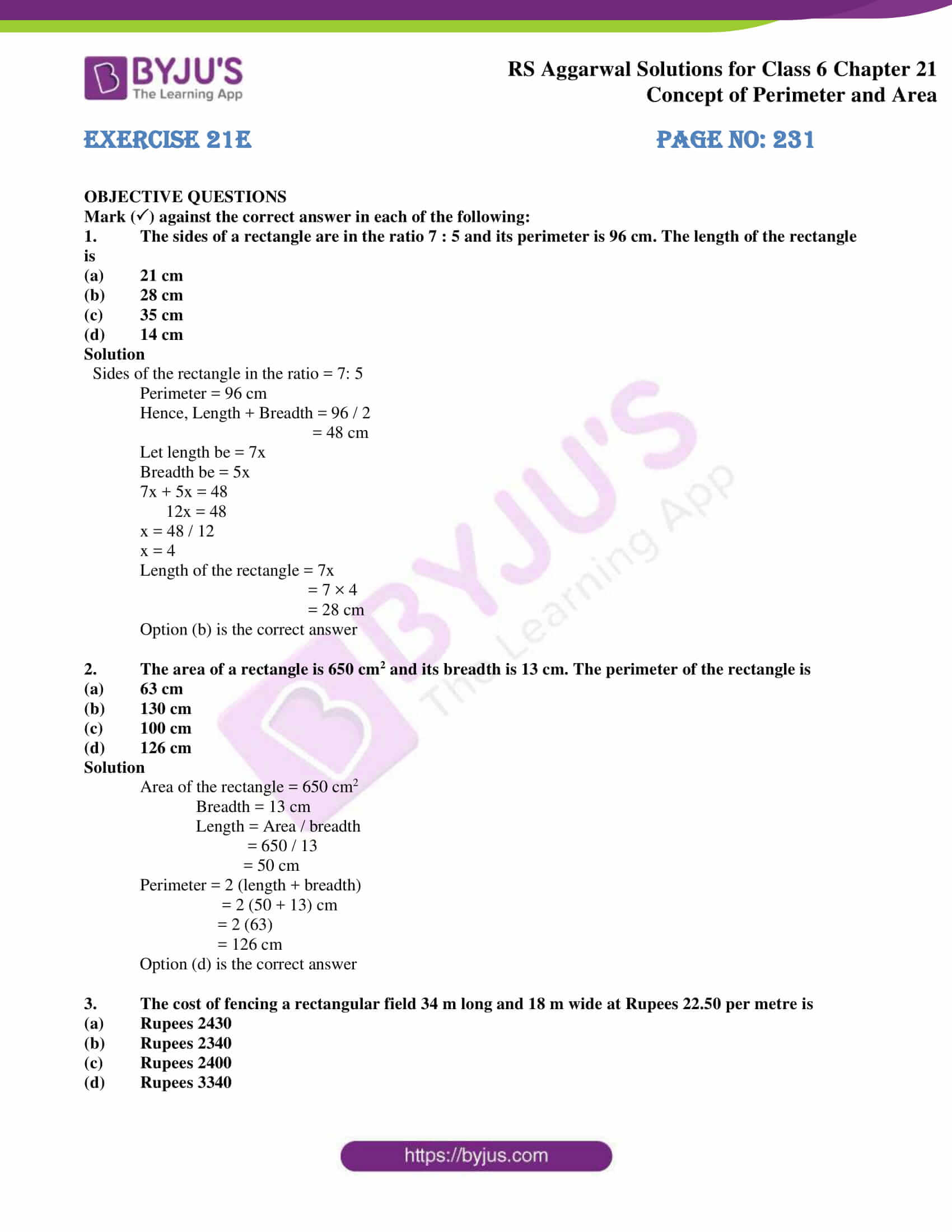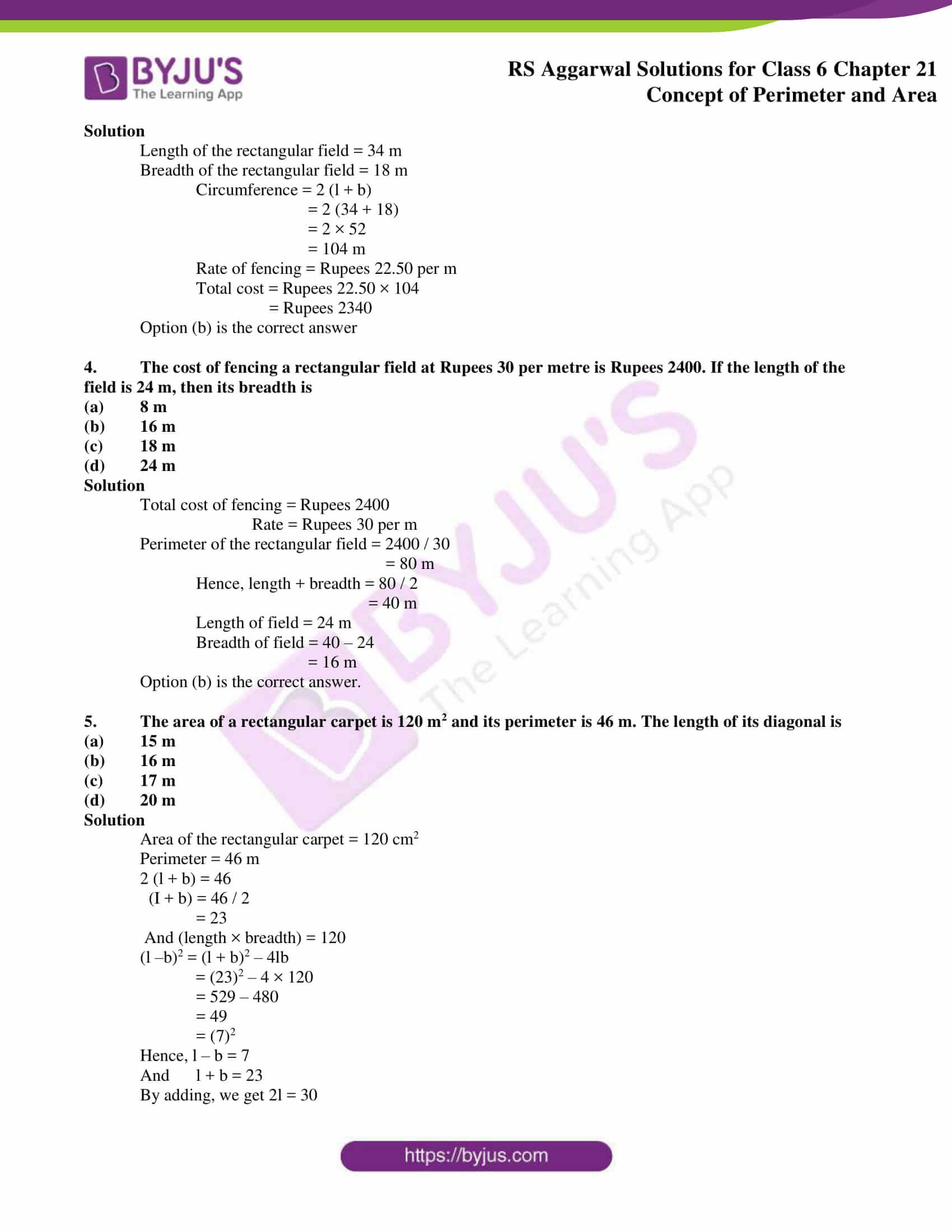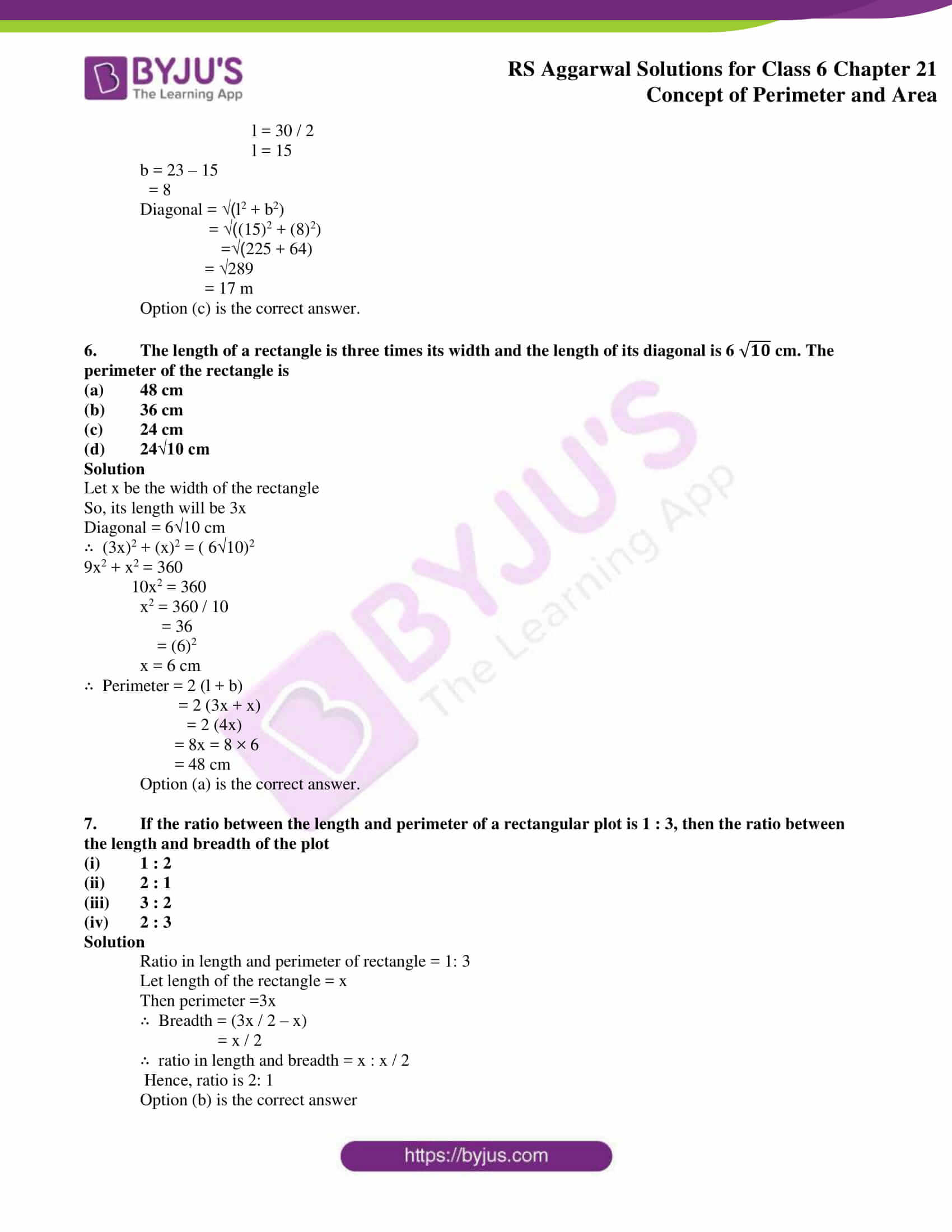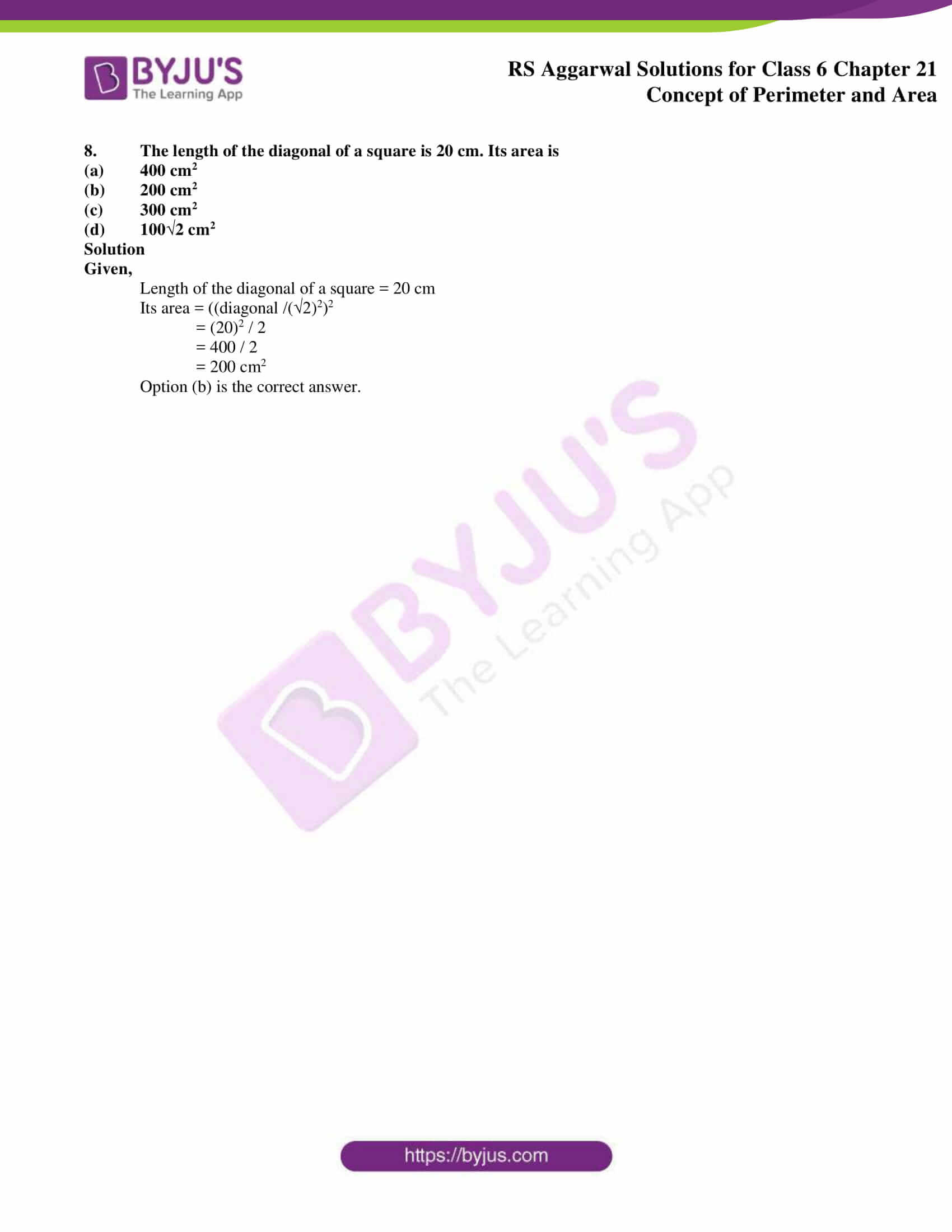# RS Aggarwal Solutions for Class 6 Chapter 21 Concept of Perimeter and Area Exercise 21E

RS Aggarwal Solutions is a vital source of reference material for the students of class 6. Exercise 21E of chapter 21 has problems which are objective type and has 4 answers among which one is the correct answer. The solutions PDF has important formulas and shortcut tips highlighted to make them simple for students during the exam preparation. RS Aggarwal Solutions for Class 6 Chapter 21 Concept of Perimeter and Area Exercise 21E are provided here.

## Download PDF of RS Aggarwal Solutions for Class 6 Chapter 21 Concept of Perimeter and Area Exercise 21E### Access answers to Maths RS Aggarwal Solutions for Class 6 Chapter 21 Concept of Perimeter and Area Exercise 21E

OBJECTIVE QUESTIONS

Mark () against the correct answer in each of the following:

1. The sides of a rectangle are in the ratio 7 : 5 and its perimeter is 96 cm. The length of the rectangle is

(a) 21 cm

(b) 28 cm

(c) 35 cm

(d) 14 cm

Solution

Sides of the rectangle in the ratio = 7: 5

Perimeter = 96 cm

Hence, Length + Breadth = 96 / 2

= 48 cm

Let length be = 7x

7x + 5x = 48

12x = 48

x = 48 / 12

x = 4

Length of the rectangle = 7x

= 7 × 4

= 28 cm

Option (b) is the correct answer

2. The area of a rectangle is 650 cm2 and its breadth is 13 cm. The perimeter of the rectangle is

(a) 63 cm

(b) 130 cm

(c) 100 cm

(d) 126 cm

Solution

Area of the rectangle = 650 cm2

= 650 / 13

= 50 cm

Perimeter = 2 (length + breadth)

= 2 (50 + 13) cm

= 2 (63)

= 126 cm

Option (d) is the correct answer

3. The cost of fencing a rectangular field 34 m long and 18 m wide at Rupees 22.50 per metre is

(a) Rupees 2430

(b) Rupees 2340

(c) Rupees 2400

(d) Rupees 3340

Solution

Length of the rectangular field = 34 m

Breadth of the rectangular field = 18 m

Circumference = 2 (l + b)

= 2 (34 + 18)

= 2 × 52

= 104 m

Rate of fencing = Rupees 22.50 per m

Total cost = Rupees 22.50 × 104

= Rupees 2340

Option (b) is the correct answer

4. The cost of fencing a rectangular field at Rupees 30 per metre is Rupees 2400. If the length of the field is 24 m, then its breadth is

(a) 8 m

(b) 16 m

(c) 18 m

(d) 24 m

Solution

Total cost of fencing = Rupees 2400

Rate = Rupees 30 per m

Perimeter of the rectangular field = 2400 / 30

= 80 m

Hence, length + breadth = 80 / 2

= 40 m

Length of field = 24 m

Breadth of field = 40 – 24

= 16 m

Option (b) is the correct answer.

5. The area of a rectangular carpet is 120 m2 and its perimeter is 46 m. The length of its diagonal is

(a) 15 m

(b) 16 m

(c) 17 m

(d) 20 m

Solution

Area of the rectangular carpet = 120 cm2

Perimeter = 46 m

2 (l + b) = 46

(I + b) = 46 / 2

= 23

And (length × breadth) = 120

(l –b)2 = (l + b)2 – 4lb

= (23)2 – 4 × 120

= 529 – 480

= 49

= (7)2

Hence, l – b = 7

And l + b = 23

By adding, we get 2l = 30

l = 30 / 2

l = 15

b = 23 – 15

= 8

Diagonal = √(l2 + b2)

= √((15)2 + (8)2)

=√(225 + 64)

= √289

= 17 m

Option (c) is the correct answer.

6. The length of a rectangle is three times its width and the length of its diagonal is 6 cm. The perimeter of the rectangle is

(a) 48 cm

(b) 36 cm

(c) 24 cm

(d) 24√10 cm

Solution

Let x be the width of the rectangle

So, its length will be 3x

Diagonal = 6√10 cm

(3x)2 + (x)2 = ( 610)2

9x2 + x2 = 360

10x2 = 360

x2 = 360 / 10

= 36

= (6)2

x = 6 cm

Perimeter = 2 (l + b)

= 2 (3x + x)

= 2 (4x)

= 8x = 8 × 6

= 48 cm

Option (a) is the correct answer.

7. If the ratio between the length and perimeter of a rectangular plot is 1 : 3, then the ratio between the length and breadth of the plot

(i) 1 : 2

(ii) 2 : 1

(iii) 3 : 2

(iv) 2 : 3

Solution

Ratio in length and perimeter of rectangle = 1: 3

Let length of the rectangle = x

Then perimeter =3x

Breadth = (3x / 2 – x)

= x / 2

ratio in length and breadth = x : x / 2

Hence, ratio is 2: 1

Option (b) is the correct answer

8. The length of the diagonal of a square is 20 cm. Its area is

(a) 400 cm2

(b) 200 cm2

(c) 300 cm2

(d) 100√2 cm2

Solution

Given,

Length of the diagonal of a square = 20 cm

Its area = ((diagonal /(√2)2)2

= (20)2 / 2

= 400 / 2

= 200 cm2

Option (b) is the correct answer.

### Access other exercises of RS Aggarwal Solutions for Class 6 Chapter 21 Concept of Perimeter and Area

Exercise 21D Solutions